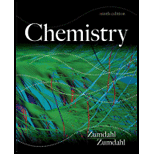# Natural gas is a mixture of hydrocarbons, primarily methane (CH 4 ) and ethane (C 2 H 6 ). A typical mixture might have χ methane = 0.915 and χ ethane = 0.085. What are the partial pressures of the two gases in a 15.00-L container of natural gas at 20.°C and 1.44 atm? Assuming complete combustion of both gases in the natural gas sample, what is the total mass of water formed?### Chemistry

9th Edition
Steven S. Zumdahl
Publisher: Cengage Learning
ISBN: 9781133611097

#### Solutions

Chapter
Section### Chemistry

9th Edition
Steven S. Zumdahl
Publisher: Cengage Learning
ISBN: 9781133611097
Chapter 5, Problem 164IP
Textbook Problem
223 views

## Natural gas is a mixture of hydrocarbons, primarily methane (CH4) and ethane (C2H6). A typical mixture might have χmethane= 0.915 and χethane = 0.085. What are the partial pressures of the two gases in a 15.00-L container of natural gas at 20.°C and 1.44 atm? Assuming complete combustion of both gases in the natural gas sample, what is the total mass of water formed?

Interpretation Introduction

Interpretation:

• The partial pressures of methane and ethane in the natural gas sample have to be calculated.
• The total mass of Water produced by the complete combustion of methane and ethane in the natural gas sample has to be determined.

Concept Introduction:

• In a mixture of gaseous substances, the total pressure of the system is equal to the sum of the partial pressure of the each gaseous substance.
• Thus, the pressure contributed by the individual gaseous substance to the total pressure is known as the partial pressure of that gaseous substance.
• The partial pressure of a gas depends upon its mole fraction. Mole fraction of a gaseous component is nothing but the number of moles of that particular gaseous substance present in a system containing mixture of gaseous substances.
• Let us assume a system contains a mixture of two different gases A and B. Let ‘N’ be the total number of moles of gases present in the mixture. Then,

Mole fraction of gas A is, XA=numberofmolesofATotalnumberofmoles

Mole fraction of gas B is, XB=numberofmolesofBTotalnumberofmoles

• Using the number of moles and mole fraction of the reactants and products, the amount of product obtained can be determined.

### Explanation of Solution

Explanation

Calculate the partial pressures of CH4 and C2H6 using their respective mole fractions.

Given data:

molefractionofmethaneχmethane=0.915molefractionofethaneχethane=0.085                      Total pressure      = 1.44 atm                         Volume            =15L

partialpressure=totalpressure×molefractiontherefore,PCH4=Ptotal×χCH4=1.44×0.915=1.32atmPC2H6=Ptotal×χC2H6=1.44×0.085=0.12atm

Determine the number of moles of the natural gas that combusted.

PV=nRTnnaturalgas=PVRT=1.44atm×15L0.0821LatmKmol×293K=0.898mol

By substituting the given values of temperature, pressure, volume and gas constant value in the ideal gas equation, total number of moles of hydrocarbons is determined.

Determine the number of moles of methane and ethane.

numberofmolesofmethane=molefractionofmethane×totalnumberofmolesofnaturalgastherefore,nCH4=0.915×0.898=0.822Similarly,nC2H4=0

### Still sussing out bartleby?

Check out a sample textbook solution.

See a sample solution

#### The Solution to Your Study Problems

Bartleby provides explanations to thousands of textbook problems written by our experts, many with advanced degrees!

Get Started

Find more solutions based on key concepts
The fiber-rich portion of the wheat kernel is the bran layer. T F

Nutrition: Concepts and Controversies - Standalone book (MindTap Course List)

If you blow across the top of an empty soft-drink bottle, a pulse of sound travels down through the air in the ...

Physics for Scientists and Engineers, Technology Update (No access codes included)

How might one detect and warn against tsunami?

Oceanography: An Invitation To Marine Science, Loose-leaf Versin